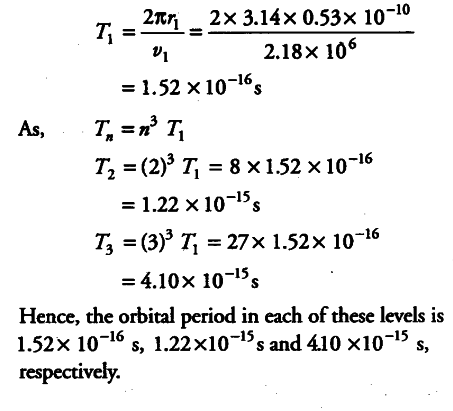# Using the Bohr’s model, calculate the speed of the electron

(i) Using the Bohr’s model, calculate the speed of the electron in a H-atom in the n = 1,2 and 3 levels.
(ii) Calculate the orbital period in each of these levels.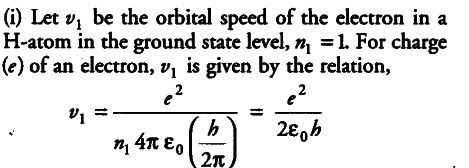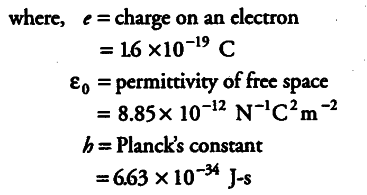{ v }_{ 1 } = 1.6 x {{10}^{-19}} x 1.6 x {{10}^{-19}} / 2 x 8.85 x {{10}^{-12}} x 6.63 x {{10}^{-34}}
= 0.0218 x {{10}^{8}} = 2.18 x {{10}^{6}} m/s
We know that { v }_{ n }=
{ v }_{ 1 } /n
and for level { n }_{ 2 } = 3, we can write the relation for the corresponding orbital speed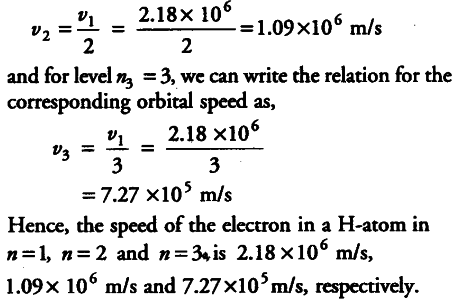(ii)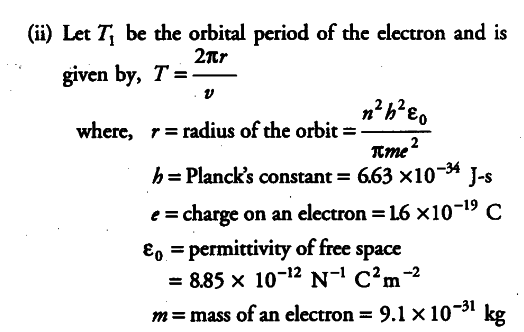For , n=1